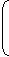# Aptitude - Banker's Discount - Discussion

### Discussion :: Banker's Discount - General Questions (Q.No.10)

10.

 The banker's gain on a certain sum due 1 1 years hence is 3 of the banker's 2 25

discount. The rate percent is:

[A].
 5 1 % 5
[B].
 9 1 % 11
[C].
 8 1 % 8
[D].
 6 1 % 6

Answer: Option B

Explanation:

Let, B.D = Re. 1.

 Then, B.G. = Re. 3 . 25T.D. = (B.D. - B.G.) = Re.1 - 3= Re. 22 . 25 25

 Sum =1 x (22/25)= Rs. 22 . 1-(22/25) 3

 S.I. on Rs. 22 for 1 1 years is Re. 1. 3 2Rate =100 x 1%
 = 100 = 9 1 %. 11 11
 22 x 3 3 2

 Arun said: (Jul 7, 2011) What is the B.D and B.G?

 King said: (Dec 15, 2011) B.D means banker discount and B.G means banker gain

 Vikram said: (Mar 29, 2012) How is possible for Re. 1.

 Ruchi said: (Sep 21, 2013) Given T = 3/2. B.G = 3/25 of B.D. R = ? Now, T.D = B.D - B.G. (True discount = Banker's discount - Banker's gain). T.D = B.D - 3/25B.D. T.D = 22/25 B.D........(1). Again TD = BD*100/(100+RT). From equ(1). 22/25 BD = BD*100 /[100+(3/2)R]. 22/25 = 100/[100+(3/2)R]. R = 100/11. Real = 9 1/11.

 Mohd Irshad said: (Apr 17, 2015) Dear, Can you explain in brief?

 Suresh said: (Nov 7, 2015) (1*22/25)/(1-22/25)? How it comes for 3/25?

 Karthika said: (Jun 27, 2016) @Suresh 1*22/25 = 55/25. 1 - 22/25 = 3/25. = 22/3.

 Surakshitha said: (Jul 19, 2016) Anyone. Please tell me a common procedure to find the rate percent.

 Swetha said: (Aug 25, 2016) Please explain the answer simply and clearly.

 Chetanpreet said: (Oct 15, 2016) T.D = B.G X 100/T X R, Assume B.D = Rs 1, Then T.D = B.D - B.G.

 Saleem Khan said: (Oct 23, 2016) How can we assume BD = 1?

 Syed said: (Feb 14, 2018) Please explain the Answer Clearly.

 Shashi said: (Nov 10, 2018) Very easy method @Ruchi, thank you.

#### Post your comments here:

Name *:

Email   : (optional)

» Your comments will be displayed only after manual approval.

#### Current Affairs 2021

Interview Questions and Answers